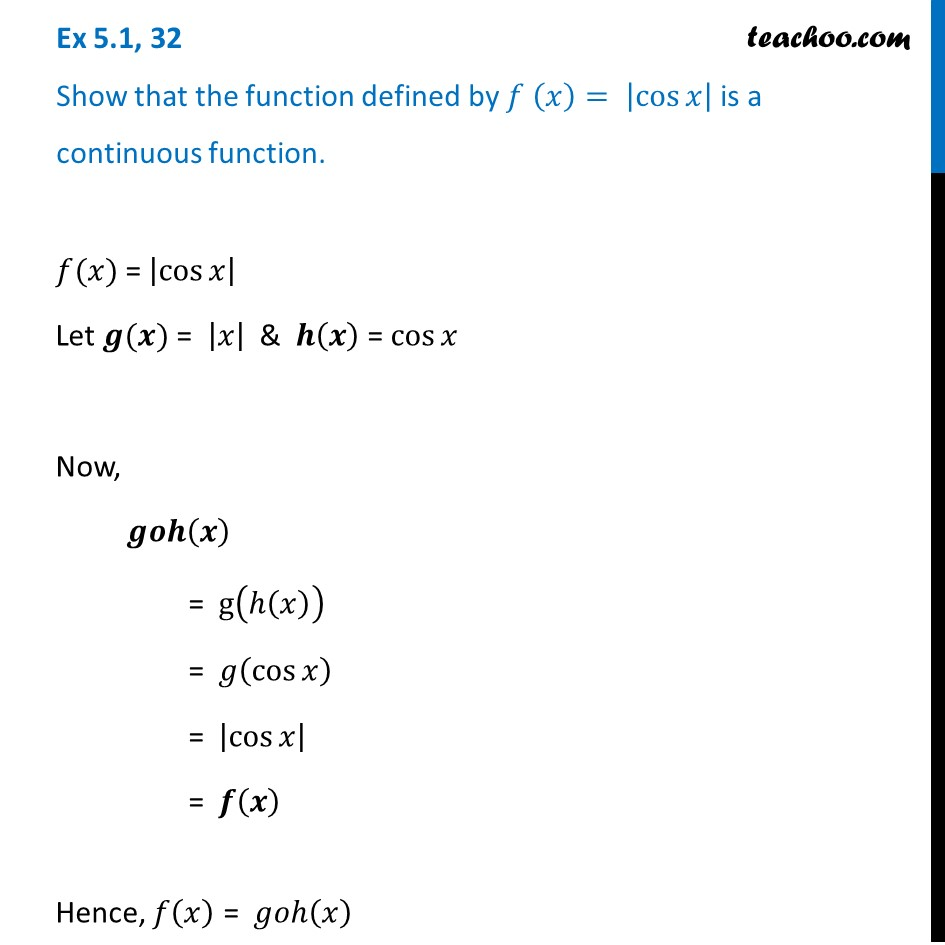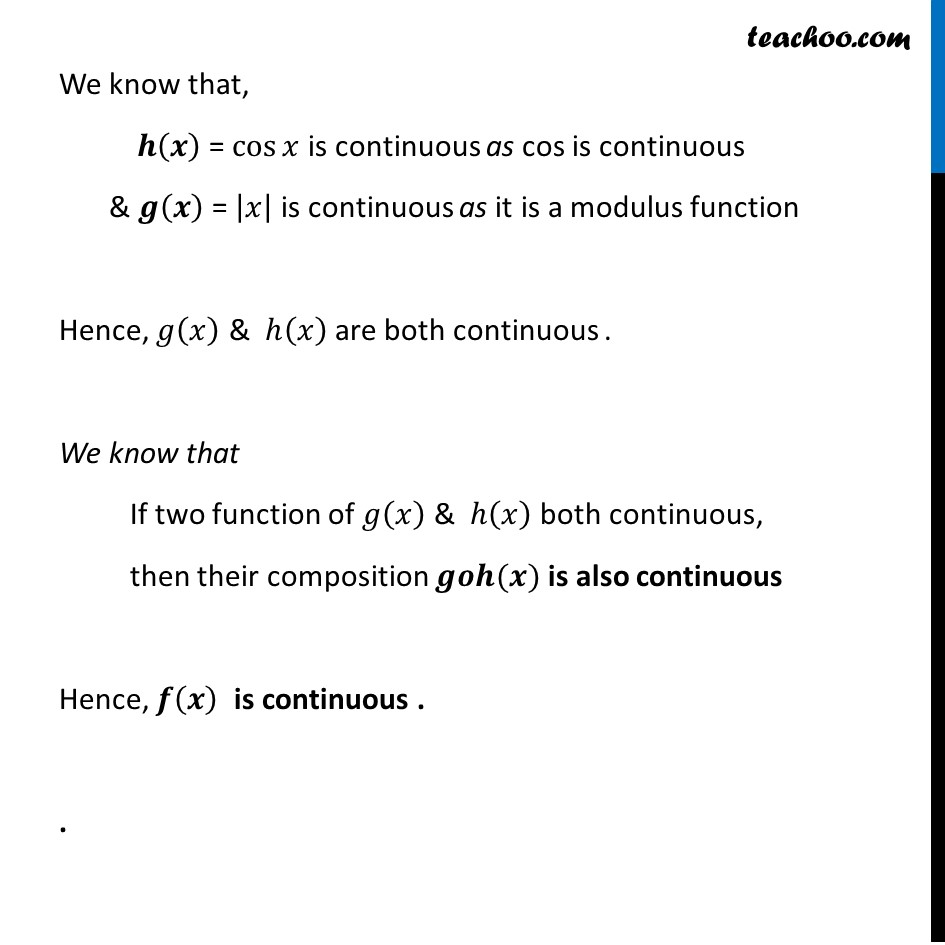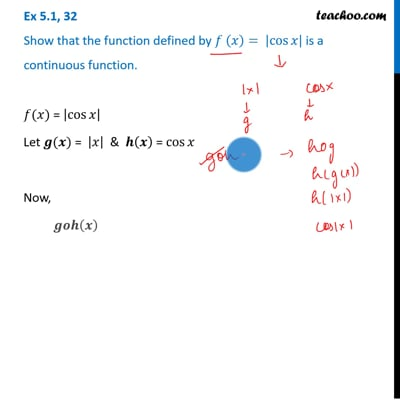Continuity of composite functions

Chapter 5 Class 12 Continuity and Differentiability
Concept wiseThis video is only available for Teachoo black users

Introducing your new favourite teacher - Teachoo Black, at only ₹83 per month

### Transcript

Ex 5.1, 32 Show that the function defined by 𝑓 (𝑥)= |cos⁡𝑥 | is a continuous function.𝑓(𝑥) = |cos⁡𝑥 | Let 𝒈(𝒙) = |𝑥| & 𝒉(𝒙) = cos⁡𝑥 Now, 𝒈𝒐𝒉(𝒙) = g(ℎ(𝑥)) = 𝑔(cos⁡𝑥 ) = |cos⁡𝑥 | = 𝒇(𝒙) Hence, 𝑓(𝑥) = 𝑔𝑜ℎ(𝑥) We know that, 𝒉(𝒙) = cos⁡𝑥 is continuous as cos is continuous & 𝒈(𝒙) = |𝑥| is continuous as it is a modulus function Hence, 𝑔(𝑥) & ℎ(𝑥) are both continuous . We know that If two function of 𝑔(𝑥) & ℎ(𝑥) both continuous, then their composition 𝒈𝒐𝒉(𝒙) is also continuous Hence, 𝒇(𝒙) is continuous . .